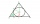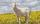# Derivation + area - math problems

#### Number of problems found: 6

• MartiansA sphere-shaped spaceship with a diameter of 6 m landed in the meadow. In order not to attract attention, the Martians covered it with a roof in the shape of a regular cone. How high will this roof be so that the consumption of roofing is minimal?
• MegapizzaMegapizza will be divided among 100 people. First gets 1%, 2nd 2% of the remainder, 3rd 3% of the remainder, etc. Last 100th 100% of the remainder. Which person got the biggest portion?
• Maximum of volumeThe shell of the cone is formed by winding a circular section with a radius of 1. For what central angle of a given circular section will the volume of the resulting cone be maximum?
• Cylindrical containerAn open-topped cylindrical container has a volume of V = 3140 cm3. Find the cylinder dimensions (radius of base r, height v) so that the least material is needed to form the container.
• Rectangle poolFind dimensions of an open pool with a square bottom with a capacity of 32 m3 to have painted/bricked walls with the least amount of material.
• GoatMeadow is a circle with a radius r = 19 m. How long must a rope tie a goat to the pin on the perimeter of the meadow to allow the goat to eat half of the meadow?

We apologize, but in this category are not a lot of examples.
Do you have an exciting math question or word problem that you can't solve? Ask a question or post a math problem, and we can try to solve it.

We will send a solution to your e-mail address. Solved examples are also published here. Please enter the e-mail correctly and check whether you don't have a full mailbox.

Derivation - math problems. Area - math problems.